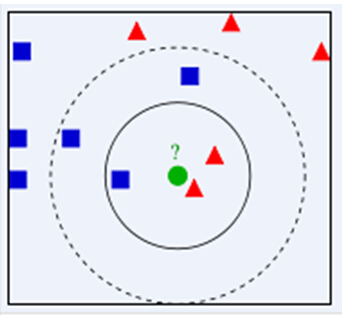《Django REST framework 入门到精通》视频课程上线了，全网稀缺资源，深度源码剖析，进阶高手之路！点击链接查看详情

# 纯Python实现kNN机器学习算法

k近邻（k-Nearest Neighbor）算法，简称kNN，可能是最简单也最好理解的监督学习方法了。• 某种距离度量方法：一般是欧氏距离。当然也可以使用其它的。
• 最靠近的：只看邻居，不看远亲。
• k个：显而易见的超参数。也就是考察的邻居数量。
• 基于邻居的信息进行预测，有不同的预测方法。分类和回归又有不同。

k是k近邻算法最重要的参数，不同的k值，可能导致完全不同的分类结果。当k为1的时候，被称为‘最近邻分类器’。

• 积极学习
• 懒惰学习

```import random
import bisect

"""

"""

def cal_distance(a, b):
"""
只计算平方和，省去开根号的计算开销。
:param a:
:param b:
:return: 欧氏距离的平方
"""

return (a-b)**2 + (a-b)**2

def kNNClassifier(train_set, k, i):
"""
构造三个包含k个元素的列表，分别用来保存最小距离、最小距离点、点对应的分类标记。
当数据集较大的时候，这个方法可能会快一点。数据集较小的时候，建议直接排序。
:param train_set: 训练集
:param k: 判定的邻居数量
:param i: 预测点
:return: 预测分类
"""

# 使用训练集的第一个元素初始化三个列表
distances = [cal_distance(train_set, i)]*k   # 从小到大排列的k个元素
points = [train_set]*k        # 与distances对应的点的列表
labels = [train_set]*k        # 与points对应的点的分类

# 遍历训练集中的每个点
for item in train_set:
dis = cal_distance(item, i)   # 计算它与点i的距离
if dis < distances[-1]:    # 如果这个距离比当前distances列表中最后一个元素的值小
index = bisect.bisect(distances, dis)   # 使用Python内置的bisect二分法，查找插入的位置
distances.insert(index, dis)   # 执行插入操作
distances.pop()     # 弹出最后一个元素，保证distances中始终只有k个元素

points.insert(index, item)   # 与上面的操作类似
points.pop()

labels.insert(index, item)   # 与上面的操作类似
labels.pop()

print('最小的距离值列表：   ', distances)
print('对应的点的列表：   ', points)
print('点的分类标记列表：   ', labels)

# 统计每类标记的数量
count_dic = {labels.count(key): key for key in set(labels)}

# 返回数量最多的标记以及对应的数量
return count_dic[max(count_dic)], max(count_dic)

if __name__ == '__main__':

# random.seed(42)  # 如果不执行这行代码，每次生成的数据集都是随机的，结果不可重现。如果定义相同的seed，则每次执行的结果可重现。

# 随机获取1万个，1-10000的整数点(x, y)
random_points = [(random.randint(1, 10000), random.randint(1, 10000)) for _ in range(10000)]

# print(points)
# print(len(points))

# 去掉可能产生的重复点，这可能导致实际训练集中点的数量小于10000.
points = set(random_points)

# print(points)
# print(len(points))

# 随机为每个点标记分类
train_data = {}

for item in points:
train_data[item] = random.choice(['A', 'B'])   # 可以自己指定分类标记和数量

# list(train_data.items())类似
# [((4879, 4728), 'B'), ((7417, 3998), 'A'), ....,((3312, 4037), 'B'), ((1944, 7900), 'A')]
predict, num = kNNClassifier(list(train_data.items()), 10, (23, 48))

print('预测分类为：', predict, '  有{}个最近邻属于该分类'.format(num))
```

#### 评论总数： 3k近邻